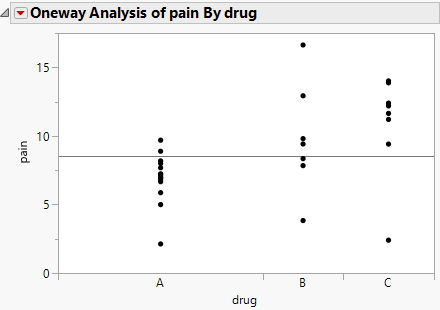Basic Analysis > Oneway Analysis
Publication date: 11/10/2021

# Oneway Analysis

##### Examine Relationships between a Continuous Y and a Categorical X Variable

Use the Oneway platform to explore how the distribution of a continuous Y variable differs across groups defined by a categorical X variable. You can use ANOVA, nonparametric tests, or multiple comparisons to evaluate differences in means across groups. Density and CDF plots enable you to visualize the distribution of your response by the X categories. For example, you might want to find out how different categories of the same type of drug (X) affect patient pain levels on a numerical scale (Y).

The Oneway platform is the continuous by nominal or ordinal personality of the Fit Y by X platform. The analysis results appear in a plot, and you can interactively add additional analyses, such as the following:

a one-way analysis of variance to fit means and to test that they are equal

nonparametric tests

a test for homogeneity of variance

multiple-comparison tests on means, with means comparison circles

outlier box plots overlaid on each group

power details for the one-way layout

Figure 6.1 Oneway Analysis